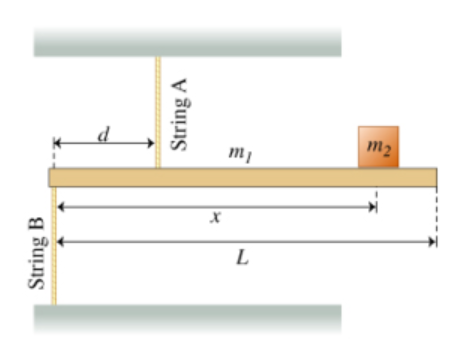# Problem: A Bar Suspended by Two Vertical Strings. The figure (Figure 1) shows a model of a crane that may be mounted on a truck. A rigid uniform horizontal bar of mass m1 = 85.0 kg and length L = 5.70 m is supported by two vertical massless strings. String A is attached at a distance d = 1.50 m from the left end of the bar and is connected to the top plate. String B is attached to the left end of the bar and is connected to the floor. An object of mass m2 = 2000 kg is supported by the crane at a distance x = 5.50 m from the left end of the bar. Throughout this problem, positive torque is counterclockwise and use 9.80 m/s2 for the magnitude of the acceleration due to gravity.Part A. Find the TA, the tension in string A. Express your answer in newtons using the three significant figures.Part B. Find the TB, the magnitude of the tension in string B. Express your answer in newtons using three significant figures.

###### FREE Expert Solution

In this problem, we'll apply the sum of torque and the sum of forces for a system in equilibrium.

Torque:

$\overline{){\mathbf{\tau }}{\mathbf{=}}{\mathbf{r}}{\mathbf{F}}}$

In equilibrium:

$\overline{){\mathbf{\Sigma }}{\mathbf{\tau }}{\mathbf{=}}{\mathbf{0}}}$

93% (422 ratings)###### Problem Details

A Bar Suspended by Two Vertical Strings. The figure (Figure 1) shows a model of a crane that may be mounted on a truck. A rigid uniform horizontal bar of mass m1 = 85.0 kg and length L = 5.70 m is supported by two vertical massless strings. String A is attached at a distance d = 1.50 m from the left end of the bar and is connected to the top plate. String B is attached to the left end of the bar and is connected to the floor. An object of mass m2 = 2000 kg is supported by the crane at a distance x = 5.50 m from the left end of the bar.

Throughout this problem, positive torque is counterclockwise and use 9.80 m/s2 for the magnitude of the acceleration due to gravity.Part A. Find the TA, the tension in string A. Express your answer in newtons using the three significant figures.

Part B. Find the TB, the magnitude of the tension in string B. Express your answer in newtons using three significant figures.